Courses

# Basic Mathematics and Measurement(Part- 2) - Physics, Solution by DC Pandey NEET Notes | EduRev

## DC Pandey (Questions & Solutions) of Physics: NEET

Created by: Ciel Knowledge

## NEET : Basic Mathematics and Measurement(Part- 2) - Physics, Solution by DC Pandey NEET Notes | EduRev

The document Basic Mathematics and Measurement(Part- 2) - Physics, Solution by DC Pandey NEET Notes | EduRev is a part of the NEET Course DC Pandey (Questions & Solutions) of Physics: NEET.
All you need of NEET at this link: NEET

Section-II
Subjective Questions

Trigonometry

Ques 1: Find the value of
(a) cos 120°
(b) sin 240°
(c) tan (-60°)
(d) cot 300°
(e) tan 330°
(f) cos (-60°)
(g) sin (-150°)
(h) cos (-120°)
(e) tan 330°
(f) cos (-60°)
(g) sin (-150°)
(h) cos (-120°)
Ans: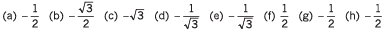Ques 2: Find the value of
(a) sec2θ - tan2θ
(b) cosec2θ - cot2θ - 1
(c) 2 sin 45°cos 45°
(d) 2sin 15°cos 45°
Ans: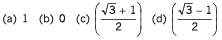Calculus

Ques 3: Differentiate the following functions with respect to x
(a) x4 + 3x2 - 2x
(b) x2 cos x
(c) (6x + 7 )4
(d) exx5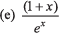Ans: (a) 4x3 + 6x - 2
(b) 2x cosx - x2 sinx
(c) 24(6x + 7)3
(d) 5exx4 + exx5
(e) -xe-x

Ques 4: Integrate the following functions with respect to t
(a) ∫(3t2 - 2t)dt
(b) ∫(4 cos t + t2) dt
(c) ∫(2t - 4)-4 dt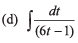Ans: (a) t3 - t2 + C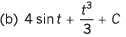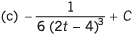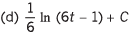Ques 5: Integrate the following functions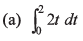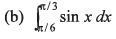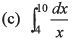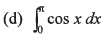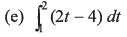Ans: (a) 4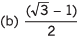(c) In (5/2)
(d) Zero
(e) - 1

Ques 6: Find maximum/minimum value of y in the functions given below
(a) y = 5 - (x - 1)2
(b) y = 4x2 - 4x + 7
(c) y = x3 - 3x
(d) y = x3 - 6x2 + 9x+ 15
(e) v = (sin 2x - x),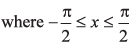Ans: (a) ymax = 5 at x = 1
(b) ymin = 6 at x = 1/2
(c) ymin = - 2 at x = 1 and ymax = 2 at x = - 1
(d) ymin = 15 at x = 3 and ymax = 19 at x = 1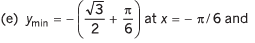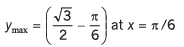Graphs

Ques 7: Draw the graphs corresponding to the equations
(a) y = 4x
(b) y = - 6x
(c) y = x + 4
(d) y = -2x + 4
(e) y = 2x - 4
(f) y = -4x - 6
Ans: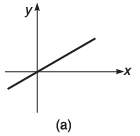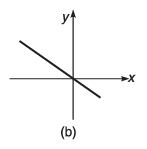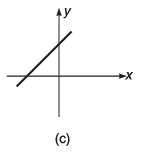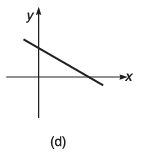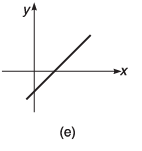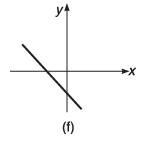Ques 8: For the graphs given below, write down their x-y equations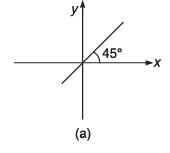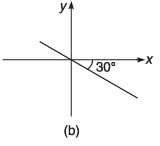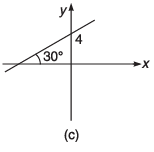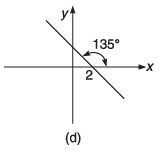Ans: (a) y = x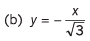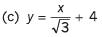(d) y = - x + 2

Ques 9: For the equations given below, tell the nature of graphs.
(a) y = 2x2
(b) y = -4x2 +6
(c) y = 6e -4x
(d) y = 4 (1 - e -2x)
(e) y = 4/x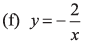Ans: (a) parabola passing through origin
(b) parabola not passing through origin
(c) exponentially decreasing graph
(d) exponentially increasing graph
(e) Rectangular hyperbola in first and third quadrant
(f) Rectangular hyperbola in second and fourth quadrant

Ques 10: Value of y decreases exponentially from y = 10 to y = 6 Plot a x-y graph.
Ans: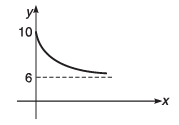Ques 11: Value of y increases exponentially from y = -4 to y = +4. Plot a x- y graph.
Ans: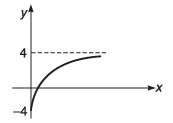Ques 12: The graph shown in figure is exponential. Write down the equation corresponding to the graph.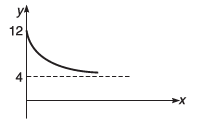Ans: y = 4 + 8e -Kx Here, K is a positive constant

Ques 13: The graph shown in figure is exponential. Write down the equation corresponding to the graph.
Ans: y = - 4 + 10(1 - e-Kx)Here, K is positive constant

Significant Figures

Ques 14: Write down the number of significant figures in the following.
(a) 6428
(b) 62.00 m
(c) 0.0628 cm
(d) 1200 N
Ans: (a) Four
(b) Four
(c) Three
(d) Four

Ques 15: Round off to four significant figures.
(a) 45.689
(b) 2.0082
Ans: (a) 45.69
(b) 2.008

Ques 16: Add 6.75 x 103 cm and 4.52x 102 cm with due regards to significant figures.
Ans: 7.20 x 10m

Ques 17: A thin wire has length of 21.7 cm and radius 0.46 mm. Calculate the volume of the wire to correct significant figures.
Ans: 0.14 cm3

Ques 18: A cube has a side of length 2.342 m. Find volume and surface area in correct significant figures.
Ans: Area= 5.485 m2, volume= 12.85 m3

Ques 19: Find density when a mass of 9.23 kg occupies a volume of 1.1m3. Take care of significant figures.
Ans: Density = 8.4 kg/m3

Ques 20: Length, breadth and thickness of a rectangular slab are 4.234 m, 1.005 m and 2.01 cm respectively. Find surface area and volume to correct significant figures.
Ans: Area= 4.255 m2, volume = 8.55 m3

Ques 21: Solve with due regards to significant figures
(4.0 x 10-4 -2.5 x 10-6)
Ans: 4.0 x 10-4

Error Analysis

Ques 22: The refractive index (n) of glass is found to have the values 1.49, 1.50, 1.52,1.54 and 1.48. Calculate
(a) the mean value of refractive index
(b) absolute error is each measurement
(c) fractional error and
(d) percentage error
Ans: (a) 1.51
(b) + 0.02,+ 0.01,- 0.01,- 0.03,+ 0.03
(c) ± 0.0132
(d) ± 1.32%

Ques 23: The radius of a sphere is measured to be (2.1 + 0.5)cm. Calculate its surface area with error limits.
Ans: (55.4±26.4) cm2

Ques 24: Calculate focal length of a spherical mirror from the following observations. Object distance u = (50.1 + 0.5) cm and image distance v = (20.1 ± 0.2) cm.
Ans: (14.3 ± 0.4) cm

Ques 25: Find the percentage error in specific resistance given by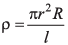where r is the radius having value (0.2+ 0.02)cm, R is the resistance of (60 ± 2) ohm and l is the length of (150 ± 0.1)cm.
Ans: 3.4%

Ques 26: A physical quantity ρ is related to four variables α, β, γ and η as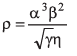The percentage errors o f measurements in α, β, γ and η are 1%, 3%, 4% and 2% respectively. Find the percentage error in ρ.
Ans: 3%

Ques 27: The period of oscillation of a simple pendulum is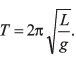Length L is about 10 cm and is known to 1 mm accuracy. The period of oscillation is about 0.5 s. The time of 100 oscillations is measured with wristwatch of 1 s time period. What is accuracy in the determination of g.
Ans: 5%

Vernier Callipers and Screw Gauge

Ques 28: 19 divisions on the main scale of a vernier callipers coincide with 20 divisions on the vernier scale. If each division on the main scale is of 1 cm, determine the least count of instrument.
Ans: 0.05 cm

Ques 29: The pitch of a screw guage is 1 mm and there are 100 divisions on the circular scale. While measuring the diameter of a wire, the linear scale reads 1 mm and 47th division on the circular scale coincides with the reference line. The length of the wire is 5.6 cm. Find the curved surface area (in cm2) of the wire in appropriate number of significant figures.
Ans: 2.6 cm2

Ques 30: The edge of a cube is measured using a vernier callipers. [9 divisions of the main scale is equal to 10 divisions of vernier scale and 1 main scale division is 1 mm]. The main scale division reading is 10 and 1 division of vernier scale was found to be coinciding with the main scale. The mass of the cube is 2.736 g. Calculate the density ing/cm3 upto correct significant figures.
Ans: 2.66 g/cm3

Offer running on EduRev: Apply code STAYHOME200 to get INR 200 off on our premium plan EduRev Infinity!

210 docs

,

,

,

,

,

,

,

,

,

,

,

,

,

,

,

,

,

,

,

,

,

,

,

,

;